### Home > CALC > Chapter 6 > Lesson 6.3.1 > Problem6-84

6-84.
1. Using your graphing calculator, evaluate the following integrals. Homework Help ✎

1.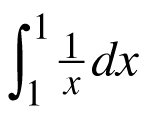2.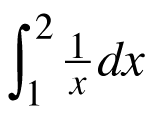3.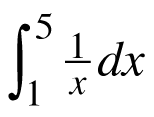4.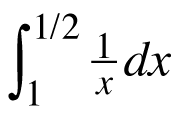5.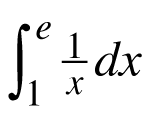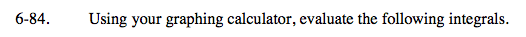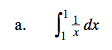Notice the bounds.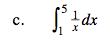Refer to part (b).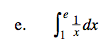This should give a pleasing value.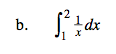$\text{Recall that if }y=\ln(x),\text{ then }y^\prime=\frac{1}{x}.$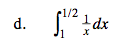Refer to part (b).